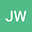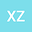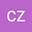Statistical Solutions for a Nonautonomous Modified Swift-Hohenberg Equation
•••Xiaoqian Zhang
Wenzhou University
Author ProfileCaidi Zhao
Wenzhou University
Author Profile## Abstract

We consider the nonautonomous modified Swift-Hohenberg equation $$u_t+\Delta^2u+2\Delta u+au+b|\nabla u|^2+u^3=g(t,x)$$ on a bounded smooth domain $\Omega\subset\R^n$ with $n\leqslant 3$. We show that, if $|b|<4$ and the external force $g$ satisfies some appropriate assumption, then the associated process has a unique pullback attractor in the Sobolev space $H_0^2(\Omega)$. Based on this existence, we further prove the existence of a family of invariant Borel probability measures and a statistical solution for this equation.
27 Apr 2021Submitted to Mathematical Methods in the Applied Sciences
28 Apr 2021Assigned to Editor
28 Apr 2021Submission Checks Completed
01 May 2021Reviewer(s) Assigned
20 Jul 2021Review(s) Completed, Editorial Evaluation Pending
21 Jul 2021Editorial Decision: Accept
Dec 2021Published in Mathematical Methods in the Applied Sciences volume 44 issue 18 on pages 14502-14516. 10.1002/mma.7719